Methods and formulas for expected overall performance in Normal Capability Analysis

PPM < LSL for expected overall performance

The parts per million less than the lower specification limit (PPM < LSL) and the percentage less than the lower specification limit (% < LSL) are both found from the probability that a part is below the specification limit (P(x < LSL)).

PPM < LSL and % < LSL are multiples of the probability:

Two-sided confidence intervals

Confidence intervals for P(x < LSL) are given by the following formulas:

where

Confidence intervals for PPM < LSL and % < LSL are found by multiplying the confidence intervals for the probability by a constant.

One-sided confidence bound

For a one-sided bound, the calculations follow:

Minitab solves the following equation to find p1:

where

Notation

Notation

TermDescription
LSLthe lower specification limit
USLthe upper specification limit
LBthe lower bound
UBthe upper bound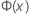the Cumulative Distribution Function (CDF) from a standard normal distribution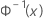the inverse CDF from a standard normal distribution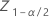the (1 - α/2)th percentile of the standard normal distribution
αthe alpha for the confidence levelthe process mean (estimated from the sample date or a historical value)
sthe sample overall standard deviation
Nthe total number of measurements
υthe degrees of freedom for s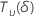a random variable that is distributed as a non-central t distribution with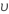degrees of freedom and non-centrality parameter δ

PPM > USL for expected overall performance

The parts per million greater than the upper specification limit (PPM > USL) and the percentage greater than the upper specification limit (% > USL) are both found from the probability that a part is above the specification limit (P(x > USL)).

PPM > USL and % > USL are multiples of the probability:

Two-sided confidence intervals

Confidence intervals for P(x > USL) are given by the following formulas:

where

Confidence intervals for PPM > USL and % > USL are found by multiplying the confidence intervals for the probability by a constant.

One-sided confidence bound

For a one-sided bound, the calculations follow:

Minitab solves the following equation to find p1:

where

Notation

TermDescription
USLthe upper specification limit
PPMthe parts per million
LBthe lower bound
UBthe upper boundthe Cumulative Distribution Function (CDF) from a standard normal distributionthe inverse CDF from a standard normal distributionthe (1 - α/2)th percentile of the standard normal distribution
αthe alpha for the confidence levelthe process mean (estimated from the sample date or a historical value)
sthe sample overall standard deviation
Nthe total number of measurements
υthe degrees of freedom for sa random variable that is distributed as a non-central t distribution withdegrees of freedom and non-centrality parameter δ

PPM Total for expected overall performance

The expected number of parts per million that are outside of the specification limits, based on the overall variation of the process, is

Notation

TermDescription
PPMParts per million
LSLLower specification limit
USLUpper specification limit
Φ (X) Cumulative distribution function (CDF) of a standard normal distributionAverage of the observations
sOverall standard deviation

Confidence intervals for PPM total for expected overall performance when both LSL and USL are known

The total Parts Per Million (PPM) out-of-specification and the percentage out-of-specification are both found from the probability that a part is outside the specification limits.

Two-sided confidence intervals

The upper and lower bounds for the probability that a part is out-of-specification have the following formulas:

where

To calculate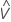, substitute the sample estimates for the parameters in the formula for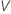:

where

The bounds for the total PPM out-of-specification and for the total percentage out-of-specification are found by multiplying the bounds for the probability by a constant.

One-sided confidence bound

The upper bound for the probability a part is out-of-specification has the following formula:

where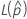andare the same as for a two-sided interval.

The upper bound for the total PPM out-of-specification and for the percentage out-of-specification are found by multiplying the bound for the probability by a constant.

Notation

TermDescription
LSLthe lower specification limit
USLthe upper specification limit
PPMthe parts per million
LBthe lower bound
UBthe upper boundthe Cumulative Distribution Function (CDF) from a standard normal distributionthe inverse CDF from a standard normal distribution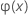the (1 - α/2)th percentile of the standard normal distribution
αthe alpha for the confidence levelthe process mean (estimated from the sample date or a historical value)
sthe sample overall standard deviation
Nthe total number of measurements
υthe degrees of freedom for sa random variable that is distributed as a non-central t distribution withdegrees of freedom and non-centrality parameter δ

Confidence intervals for PPM total for expected overall performance for a process with one specification limit

For a process with only a Lower Specification Limit (LSL), the confidence interval for the total PPM or the total percentage out of specification is the same as the confidence interval for the PPM < LSL or % < LSL. Go to the section PPM < LSL for expected overall performance.

For a process with only an Upper Specification Limit (USL), the confidence interval for the total PPM or the total percentage out of specification is the same as the confidence interval for the PPM > USL or % > USL. Go to the section on PPM > USL for expected overall performance.

By using this site you agree to the use of cookies for analytics and personalized content.  Read our policy